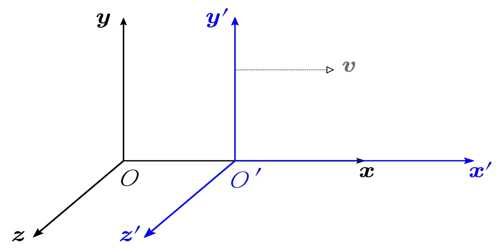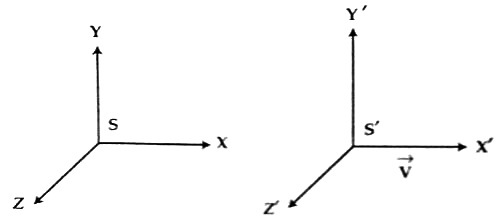# Frame of Reference

Frame of Reference

In classical and Newtonian mechanics three fundamental quantities have been considered. These are space, time, and mass. According to classical mechanics space, time and mass are constant but according to Einstein these are not absolute – all are relative. This theory of Einstein is known as the theory of relativity.

In order to describe the position or motion of a body, we need a frame of reference with respect to which it can be indicated whether the body is at rest or in motion. With reference to a nearby or distant point, a point can be determined in two or three-dimensional space. It is called a frame of reference. It is a system of geometric axes in relation to which measurements of size, position, or motion can be made. For instance, the location of a ceiling fan can be determined by taking one corner of the room as the origin and then by measuring with a scale or a tape along the length, width and height position of the fan can be fixed. Suppose the fan is fixed by measuring 3 m along the length, 2 m along the width and 3m along the height of the room. So, the coordinate of the fan is (3, 2, 3). But it is with respect to that origin. Again, if any other point inside or outside the room is taken as the origin, then the co-ordinate will be different. Most convenient and familiar frame of reference is the Cartesian coordinate system. In this system, the position of an object is determined by three mutually perpendicular axes X, Y, and Z. The position of the fan in the above example has been determined by this method. In addition to the Cartesian coordinate system, a frame of reference can be of different types like spherical cylindrical coordinates to determine the position of objects.Fig: Frame of Reference